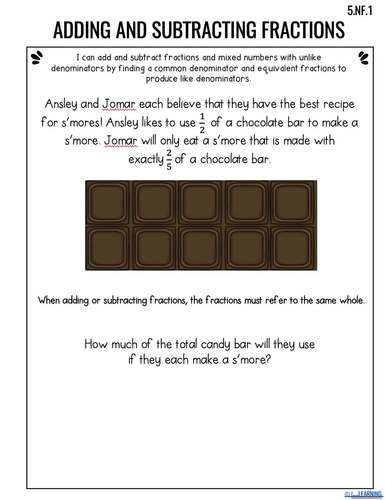# 5th Grade Math Interactive Notebook: All Fractions Standards

Rated 4.88 out of 5, based on 68 reviews
68 Ratings;
5th
Subjects
Resource Type
Standards
Formats Included
• PDF
Pages
25 Student Pages
Report this resource to TPT
Easel Activity Included
This resource includes a ready-to-use interactive activity students can complete on any device.  Easel by TPT is free to use! Learn more.

#### What educators are saying

My students loved using this resource! They were engaged in the resource and had no trouble getting started.
Any visuals or anything hands on to use with fractions is always a good resource to use in the classroom.
##### Also included in
1. These 5th grade math interactive notebooks are the perfect way to teach math to your 5th graders!This notebook includes over 100 pages that cover all 5th grade Common Core Standards.Don't like to have your students cut and glue? No worries - these notes can also be stapled or placed into math folder
Price \$18.50Original Price \$30.00Save \$11.50
2. This 5th Grade Math Bundle will completely simplify the way that you teach math for 5th grade this year! Every lesson is scaffolded and builds upon prior knowledge.Each unit includes several resources to coincide with the PowerPoint lessons. All of the lessons correlate with the resources and the in
Price \$130.00Original Price \$253.50Save \$123.50

### Description

This 5th Grade Math Interactive Notebook is perfect for teaching students about fractions. It includes notes for fractions as division, adding and subtracting fractions, multiplying fractions, and dividing fractions.

These Interactive Math Notes Cover the Following Topics:

1. Add and Subtract Fractions with Unlike Denominators 5.NF.A.1
2. Solve Word Problems 5.NF.A.2
3. Interpret Fractions as Division 5.NF.B.3
4. Multiply a Fraction by a Fraction or Whole Number 5.NF.B.4
5. Find the Area of a Rectangle Using Fractional Side Lengths 5.NF.B.4.B
6. Interpret Multiplication as Scaling 5.NF.B.5
7. Divide Unit Fractions and Whole Numbers 5.NF.B.7

This Interactive Notebook Includes:

• Cover Pages
• 2 Different Options (see preview)
• 25 pages of notes
• Simple Cut Lines to make cutting and gluing faster!
• I have also included an EASEL version that you can assign via Google Classroom™! My students used this version when we were distance learning and it worked SO well! They can easily type text or draw on their notes.

These Notes Can Also Be Found in the Following Units:

Do you want your students to Love ❤️ Learning Math?

Check out these other resources:

SAVE with Bundles:

Let's get to know each other!

2. Learn New Classroom Ideas on my Blog
4. If you have any questions, please email me: lovelearningtpt@gmail.com

Purchasing this product grants permission for use by one teacher in his or her own classroom or for one homeschool parent teaching his/her child. If you would like to share with others, please purchase additional licenses.

Total Pages
25 Student Pages
Included
Teaching Duration
2 months
Report this resource to TPT
Reported resources will be reviewed by our team. Report this resource to let us know if this resource violates TPT’s content guidelines.

### Standards

to see state-specific standards (only available in the US).
Add and subtract fractions with unlike denominators (including mixed numbers) by replacing given fractions with equivalent fractions in such a way as to produce an equivalent sum or difference of fractions with like denominators. For example, 2/3 + 5/4 = 8/12 + 15/12 = 23/12. (In general, 𝘢/𝘣 + 𝘤/𝘥 = (𝘢𝘥 + 𝘣𝘤)/𝘣𝘥.)
Solve word problems involving addition and subtraction of fractions referring to the same whole, including cases of unlike denominators, e.g., by using visual fraction models or equations to represent the problem. Use benchmark fractions and number sense of fractions to estimate mentally and assess the reasonableness of answers. For example, recognize an incorrect result 2/5 + 1/2 = 3/7, by observing that 3/7 < 1/2.
Interpret a fraction as division of the numerator by the denominator (𝘢/𝘣 = 𝘢 ÷ 𝘣). Solve word problems involving division of whole numbers leading to answers in the form of fractions or mixed numbers, e.g., by using visual fraction models or equations to represent the problem. For example, interpret 3/4 as the result of dividing 3 by 4, noting that 3/4 multiplied by 4 equals 3, and that when 3 wholes are shared equally among 4 people each person has a share of size 3/4. If 9 people want to share a 50-pound sack of rice equally by weight, how many pounds of rice should each person get? Between what two whole numbers does your answer lie?
Express a fraction with denominator 10 as an equivalent fraction with denominator 100, and use this technique to add two fractions with respective denominators 10 and 100. For example, express 3/10 as 30/100, and add 3/10 + 4/100 = 34/100.
Use decimal notation for fractions with denominators 10 or 100. For example, rewrite 0.62 as 62/100; describe a length as 0.62 meters; locate 0.62 on a number line diagram.